# Parametrix method

(diff) ← Older revision | Latest revision (diff) | Newer revision → (diff)

One of the methods for studying boundary value problems for differential equations with variable coefficients by means of integral equations.

Suppose that in some regionof the-dimensional Euclidean spaceone considers an elliptic differential operator (cf. Elliptic partial differential equation) of order,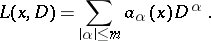(1)

In (1) the symbolis a multi-index,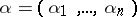, where the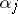are non-negative integers,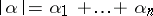,,. With every operator (1) there is associated the homogeneous elliptic operator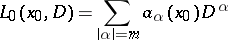with constant coefficients, where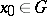is an arbitrary fixed point. Let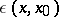denote a fundamental solution ofdepending parametrically on. Then the functionis called the parametrix of the operator (1) with a singularity at.

In particular, for the second-order elliptic operator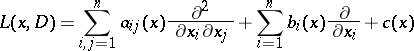one can take as parametrix with singularity atthe Levi function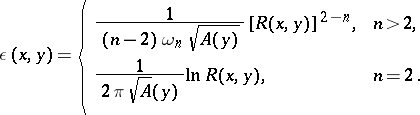(2)

In (2),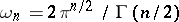,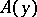is the determinant of the matrix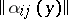,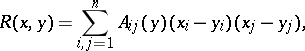and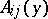are the elements of the matrix inverse to.

Letbe the integral operator(3)

acting on functions from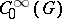and letSince, by definition of a fundamental solution,whereis the identity operator, one has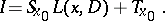This equality indicates that for every sufficiently-smooth functionof compact support inthere is a representation(4)

Moreover, ifthenis a solution of the equation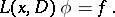Thus, the question of the local solvability of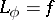reduces to that of invertibility of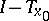.

If one applies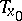to functionsthat vanish outside a ball of radiuswith centre at, then for a sufficiently smallthe norm ofcan be made smaller than one. Then the operatorexists; consequently, also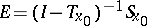exists, which is the inverse operator to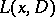. Hereis an integral operator with as kernel a fundamental solution of.

The name parametrix is sometimes given not only to the function, but also to the integral operator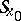with the kernel, as defined by (3).

In the theory of pseudo-differential operators, instead ofa parametrix ofis defined as an operatorsuch that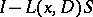and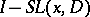are integral operators with infinitely-differentiable kernels (cf. Pseudo-differential operator). If only(or) is such an operator, thenis called a left (or right) parametrix of. In other words,in (4) is a left parametrix ifin this equality has an infinitely-differentiable kernel. Ifhas a left parametrix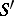and a right parametrix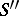, then each of them is a parametrix. The existence of a parametrix has been proved for hypo-elliptic pseudo-differential operators (see ).

How to Cite This Entry:
Parametrix method. Encyclopedia of Mathematics. URL: http://encyclopediaofmath.org/index.php?title=Parametrix_method&oldid=16020
This article was adapted from an original article by Sh.A. Alimov (originator), which appeared in Encyclopedia of Mathematics - ISBN 1402006098. See original article